# A Filter Primer

### Abstract

This comprehensive article covers all aspects of analog filters. It first addresses the basic types: first- and second-order filters, highpass and lowpass filters, notch and all-pass filters, and high-order filters. The tutorial then explains the characteristics of the different implementations, such as Butterworth filters, Chebychev filters, Bessel filters, elliptic filters, state-variable filters, and switched-capacitor filters.

### Introduction

Ease of use makes integrated, switched-capacitor filters attractive for many applications. This article helps you prepare for such designs by describing the filter products and explaining the concepts that govern their operation.

Starting with a simple integrator, we first develop an intuitive approach to active filters in general. We then introduce practical realizations such as the state-variable filter and its implementation in switched-capacitor form. Specific integrated filters described here include Maxim's MAX7400 family of higher-order switched-capacitor filters.

### First-Order Filters

#### Integrator Filters

An integrator (Figure 1a) is the simplest filter mathematically, and it forms the building block for most modern integrated filters. Consider what we know intuitively about an integrator. If you apply a DC signal at the input (i.e., zero frequency), the output will describe a linear ramp that grows in amplitude until limited by the power supplies. Ignoring that limitation, the response of an integrator at zero frequency is infinite, which means that it has a pole at zero frequency. (A pole exists at any frequency for which the transfer function's value becomes infinite.)

We also know that the integrator's gain diminishes with increasing frequency, and that at high frequencies the output voltage becomes virtually zero. Gain is inversely proportional to frequency, so it has a slope of -1 when plotted on log/log coordinates (i.e., -20dB/decade on a Bode plot, Figure 1b).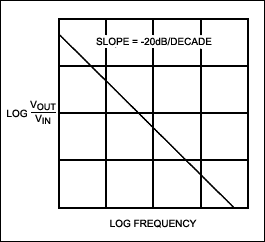You can easily derive the transfer function as:

VOUT/VIN = XC/R = (1/sC)/R = -1/(sCR) = -ω0/s

Where s is the complex-frequency variable σ + jω and ω0 is 1/RC. If we think of s as frequency, this formula confirms the intuitive feeling that gain is inversely proportional to frequency. We will return to integrators later, when discussing the implementation of actual filters.

#### Simple RC Lowpass Filters

A slightly more complex filter is the simple lowpass RC type (Figure 2a). Its characteristic (transfer function) is:

VOUT/VIN = (1/sC)/(R + 1/sC) = 1/(1 + sCR) = ω0/(s + ω0)

When s = 0, the function reduces to ω00, i.e., Unity. When s increases to infinity, the function approaches zero, so this is a lowpass filter. When s = -ω0, the denominator is zero and the function's value is infinite, indicating a pole in the complex frequency plane. The magnitude of the transfer function is plotted against s in Figure 2b, where the real component of s, σ, is toward us and the positive imaginary part, jω, is toward the right. The pole at -ω0 is evident. Amplitude is shown logarithmically to emphasize the function's form. For both the integrator and the RC lowpass filter, frequency response tends to zero at infinite frequency. Simply put, there is a zero at s = ∞. This single zero surrounds the complex plane.But how does the complex function in s relate to the circuit's response to actual frequencies? When analyzing the response of a circuit to AC signals, we use the expression jωL for the impedance of an inductor and 1/jωC for that of a capacitor. When analyzing transient response using Laplace transforms, we use sL and 1/sC for the impedance of these elements. The similarity is apparent immediately. The jω in AC analysis is, in fact, the imaginary part of s, which, as mentioned earlier, is composed of a real part s and an imaginary part jω.

If we replace s by jω in any of the above equations, we have the circuit's response to an angular frequency, ω. In the complex plot in Figure 2b, σ = 0 and hence s = jω along the positive jω axis. Thus, the function's value along this axis is the frequency response of the filter. We have sliced the function along the jω axis and emphasized the RC lowpass filter's frequency-response curve by adding a heavy line for function values along the positive jω axis. The more familiar Bode plot (Figure 2c) looks different in form only because the frequency is expressed logarithmically.While the complex frequency's imaginary part, jω, helps describe a response to AC signals, the real part, σ, helps describe a circuit's transient response. Looking at Figure 2b, we can therefore say something about the RC lowpass filter's response compared to that of the integrator. The lowpass filter's transient response is more stable, because its pole is in the negative real half of the complex plane. Restated, the lowpass filter makes a decaying-exponential response to a step-function input; the integrator makes an infinite response. For the lowpass filter, pole positions further down the -σ axis mean a higher ω0, a shorter time constant, and therefore a quicker transient response. Conversely, a pole closer to the jω axis causes a longer transient response.

So far, we have related the mathematical transfer functions of some simple circuits to their associated poles and zeroes in the complex-frequency plane. From these functions, we have derived the circuit's frequency response (and hence its Bode plot) and also its transient response. Because both the integrator and the RC filter have only one s in the denominator of their transfer functions, they each have only one pole. That is, they are first-order filters.

However, as we can see from Figure 1b, the first-order filter does not provide a very selective frequency response. To tailor a filter more closely to application needs, we must move to higher orders. From now on, we will describe the transfer function using f(s) rather than the cumbersome VOUT/VIN.

### Second-Order Lowpass Filters

A second-order filter has s2 in the denominator and two poles in the complex plane. You can obtain such a response by using inductance and capacitance in a passive circuit or by creating an active circuit of resistors, capacitors, and amplifiers. Consider the passive LC filter in Figure 3a, for example. We can show that its transfer function has the form:

ƒ(s) = XC/(R + XL + XC) = (1/sC)/[R + sL + (1/sC)] = 1/(LCS2 + RCS + 1)

If we define:

ω02 = 1/LC and Q = ω0L/R = 1/(RCω0)

Then:

ƒ(s) = 1/[(s/ω0)2 + s/(ω0Q) + 1] = ω02/[s2 + s(ω0/Q) + ω02]

Where ω0 is the filter's characteristic frequency and Q is the quality factor (lower R means higher Q).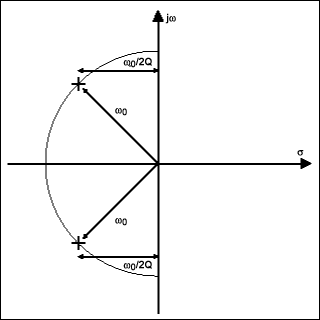The poles occur at s values for which the denominator becomes zero; that is, when s2 + sω0/Q + ω02 = 0. We can solve this equation by remembering that the roots of ax2 + bx + c = 0 are given by: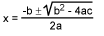In this case, a = 1, b = ω0/Q, and c = ω02. The term (b2 - 4ac) equals ω02(1/Q2 - 4). So if Q is less than 0.5 then both roots are real and lie on the negative-real axis. The circuit's behavior is much like that of two first-order RC filters in cascade. This case is not very interesting, so we will consider only the case where Q > 0.5, which means that (b2 - 4ac) is negative and the roots are complex.

The real part is therefore -b/2a, which is -ω0/2Q, and common to both roots. The roots' imaginary parts will be equal and opposite in signs. Calculating the position of the roots in the complex plane, we find that they lie at a distance of ω0 from the origin, as shown in Figure 3b. (The associated mathematics, which are straightforward but tedious, will be left as an exercise for the more masochistic readers.)

Varying ω0 changes the poles' distance from the origin. Decreasing the Q moves the poles toward each other; increasing the Q moves the poles in a semicircle away from each other and toward the jω axis. When Q = 0.5, the poles meet at -ω0 on the negative-real axis. In this case, the corresponding circuit is equivalent to two cascaded first-order filters, as noted earlier.

Now we should examine the second-order function's frequency response and see how it varies with Q. As before, Figure 4a shows the function as a curved surface, depicted in the three-dimensional space formed by the complex plane and a vertical magnitude vector. Further, Q = 0.707, and you can see immediately that the response is a lowpass filter.Increasing the Q moves the poles in a circular path toward the jω axis. Figure 4b shows the case where Q = 2. Because the poles are closer to the jω axis, they have a greater effect on the frequency response, causing a peak at the high end of the passband.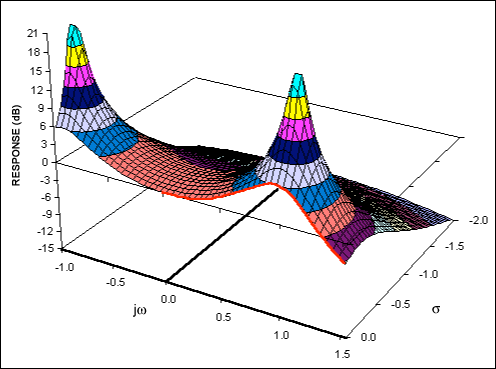There is also an effect on the filter's transient response. Because the poles' negative-real part is smaller, an input step function will cause ringing at the filter output. Lower values of Q result in less ringing, because the damping is greater. If Q becomes infinite, the poles reach the jω axis, causing an infinite frequency response (instability and continuous oscillation) at s = ω0. In the LCR circuit in Figure 3a, this condition would not be possible unless R = 0. For filters that contain amplifiers, however, the condition is indeed possible and must be considered in the design process.

A second-order filter provides the variables ω0 and Q, which allow us to place poles wherever we want in the complex plane. These poles must, nonetheless, occur as complex-conjugate pairs, in which the real parts are equal and the imaginary parts have opposite signs. This flexibility in pole placement is a powerful tool, making the second-order stage a useful component in many switched-capacitor filters. As in the first-order case, the second-order lowpass transfer function approaches zero as frequency increases to infinity. The second-order function decreases twice as fast, however, because of the s2 factor in the denominator. The result is a double zero at infinity.

Having discussed first- and second-order lowpass filters, we now need to extend our concepts in two directions: we will discuss other filter configurations, such as highpass and bandpass sections, and then we will address higher-order filters.

### Highpass and Bandpass Filters

To change a lowpass filter into a highpass filter, we turn the s plane inside out, making low frequencies high and high frequencies low. The double zero at infinite frequency goes to zero frequency; the finite response at zero frequency becomes infinite. To accomplish this transformation, we make s = ω02/s, so that s∞ when ω02/s0, and vice versa. At ω0 the old and new values of s are identical. The double zero that was at s = 1 moves to zero; the finite response that we had at s = 0 moves to infinity, producing a highpass filter:

ƒ(s) = ω02/[(ω04/s2) + (ω03/Qs) + ω02]

If we multiply the numerator and the denominator by s202,

ƒ(s) = s2/[s2 + (sω0/Q) + ω02]

This form is the same as before, except that the numerator is s2 instead of ω02. In other words, we can transform a lowpass function into a highpass one by changing the numerator and leaving the denominator alone.

The Bode plot offers another perspective on lowpass-to-highpass transformations. Figure 5a shows the Bode plot of a second-order lowpass function: flat to the cutoff frequency, then decreasing at -40dB/decade. Multiplying by s2 adds a +40dB/decade slope to this function. The additional slope provides a low-frequency rolloff below the cutoff frequency; above cutoff it gives a flat response (Figure 5b) by canceling the original -40dB/decade slope.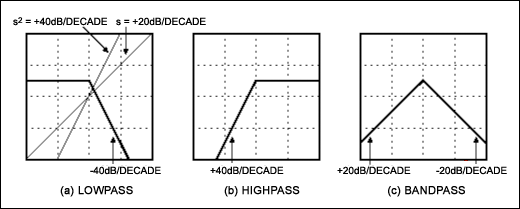We can use the same idea to generate a bandpass filter. Multiply the lowpass responses by s, which adds a +20dB/decade slope. The net response is then +20dB/decade below the cutoff and -20dB/decade above. This yields the bandpass response in Figure 5c:

ƒ(s) = ω0s/[s2 + (sω0)/Q) + ω02]

Notice that the rate of cutoff in a second-order bandpass filter is half that of the other types. This is because the available 40dB/decade slope must be shared between the two skirts of the filter.

In summary, second-order lowpass, bandpass, and highpass functions in normalized form have the same denomination, but they have numerators of ω02, ω0s, and s2, respectively.

### Notch and All-Pass Filters

A notch, or bandstop, filter rejects frequencies in a certain band while passing all others. Again, you derive this filter's transfer function by changing the numerator of the standard second-order characteristic:

ƒ(s) = (s2 + ωZ2)/s2 + (sω0/Q) + ω02

Consider the limit cases. When s = 0, f(s) reduces to ωz202, which is finite. When s∞, the equation reduces to 1. At s = jωz, the numerator becomes zero, f(s) becomes zero (a double zero, in fact, because of s2 in the numerator), and we have the characteristic of a notch filter. The gain at frequencies above and below the notch will differ unless ωz = ω0. The notch filter equation can also be expressed as:

ƒ(s) = s2 + (ωZ2)/s2 + (sω0/Q) + ω02 =
[s2/s2 + (sω0/Q) + ω02] + [ωZ2/s2 + (sω0/Q) + ω02]

This can be stated simply. The notch filter is based on the sum of a lowpass and a highpass characteristic. We use this fact in practical filter implementations to generate the notch response from existing highpass and lowpass responses. It may seem odd that we create a zero by adding two responses, but their phase relationships make it possible.

Finally, there is the all-pass filter, which has the form:

ƒ(s) = [s2 - (sω0/Q) + ω02]/[s2 + (sω0/Q) + ω02]

This response has poles and zeros placed symmetrically on either side of the jω axis, as shown in Figure 6. The effects of these poles and zeroes cancel exactly to give a level and uniform frequency response. It might seem that a piece of wire could provide this effect more cheaply. However, unlike a wire, the all-pass filter offers a useful variation of phase response with frequency.### Higher-Order Filters

We are fortunate in not having to treat the higher-order filters separately, because a polynomial in s of any length can be factored into a series of quadratic terms (plus a single first-order term if the polynomial is odd). A fifth-order lowpass filter, for instance, might have the transfer function:

ƒ(s) = 1/[s5 + a4s4 + a3s3 + a2s2 + a1s + a0]

Where all the a0 are constants. We can factor the denominator as:

ƒ(s) = 1/[(s2 + sω1/Q1 + ω12)(s2 + sω2/Q2 + ω22)(s + ω3)]

Which is the same as:

ƒ(s) = [1/(s2 + sω1/Q1 + ω12)] × [1/(s2 + sω2/Q2 + ω22)] × [1/(s + ω3)]

The last equation represents a filter that we can realize physically as two second-order sections and one first-order section, all in cascade.

This configuration simplifies the design by making it easier to visualize the response in terms of poles and zeroes in the complex-frequency plane. We know that each second-order term contributes one complex-conjugate pole pair, and that the first-order term contributes one pole on the negative-real axis. If the transfer function has a higher-order polynomial in the numerator, that polynomial can be factored as well, which means that the second-order sections will be something other than lowpass sections.

Using the synthesis principles described above, we can build a great variety of filters simply by placing poles and zeroes at different positions in the complex-frequency plane. Most applications require only a restricted number of these possibilities, however. For them, many earlier experimenters such as Butterworth and Chebychev have already worked out the details.

### The Butterworth Filter

A type of filter, common to many applications, requires a response that is flat in the passband but cuts off as sharply as possible afterwards. You can obtain that response by arranging the poles of a lowpass filter with equal spacing around a semicircular locus. The result will be a Butterworth filter. The pole-zero diagram of Figure 7a, for example, represents a fourth-order type of Butterworth filter.The poles in Figure 7a have different Q values, but they all have the same ω0 because they are the same distance from the origin. The three-dimensional surface corresponding to this filter (Figure 7b) illustrates how, as the effect of the lowest-Q pole starts to wear off, the next pole takes over, and the next, until you run out of poles and the response falls off at -80dB/decade.You can build Butterworth versions of highpass, bandpass, and other filter types, but the poles of these filters will not be arranged in a simple semicircle. In most cases, you begin by designing a lowpass filter and then applying transformations to generate the other types (such as the s1/s that we used earlier to change a lowpass into a highpass filter).

### The Chebychev Filter

By bringing poles closer to the jω axis (increasing their Qs), we can make a filter whose frequency cutoff is steeper than that of a Butterworth. This arrangement has a penalty: the effects of each pole will be visible in the filter response, giving a variation in amplitude known as ripple in the passband. With proper pole arrangement, the variations can be made equal, however, which results in a Chebychev filter.

You derive a Chebychev filter from a Butterworth by moving each pole closer to the jω axis in the same proportion, so that the poles lie on an ellipse (Figure 8a). Figure 8b demonstrates how each pole contributes one peak to the passband ripple. Moving the poles closer to the jω axis increases the passband ripple but provides a more abrupt cutoff in the stopband. The Chebychev filter, therefore, offers a trade-off between ripple and cutoff. In this respect, the Butterworth filter, in which passband ripple has been set to zero is a special case of the Chebychev.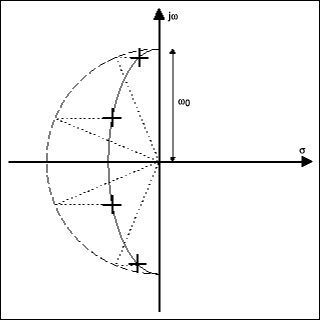### The Bessel Filter

Butterworth and Chebychev filters with sharp cutoffs carry a penalty that is evident from the positions of their poles in the s plane. Bringing the poles closer to the jω axis increases their Q, which degrades the filter's transient response. Overshoot or even ringing at the response edges can result.

The Bessel filter represents a trade-off in the opposite direction from the Butterworth. The Bessel's poles lie on a locus further from the jω axis (Figure 9). Transient response is improved, but at the expense of a less steep cutoff in the stopband.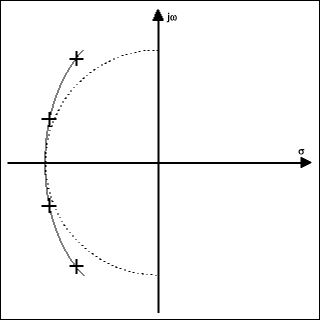### The Elliptic Filter

By increasing the Q of poles nearest the passband edge, you can obtain a filter with sharper stopband cutoff than that of the Chebychev, without incurring more passband ripple. Doing this alone would produce a gain peak, but you can compensate for the peak by providing a zero at the bottom of the stopband. Additional zeroes must be spaced along the stopband to ensure that the filter response remains below the desired level of stopband attenuation. Figure 10a shows the pole-zero diagram for this type: an elliptic filter. Figure 10b shows the corresponding transfer-function surface. As you may imagine, the elliptic filter's high-Q poles produce a transient response that is even worse than that of the Chebychev.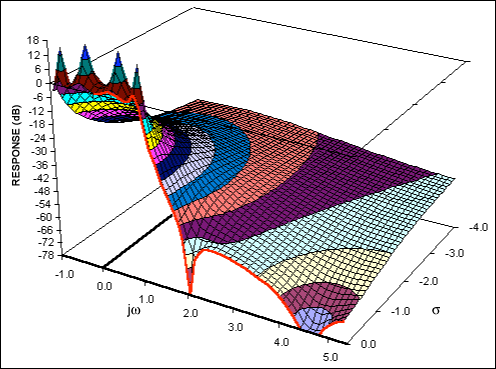Note that all the filters described have the same number of zeros as poles. (This must be the case, or the transfer function would not be a dimensionless expression.) Elliptic filters, for example, space their zeroes along the jω axis in the stopband. In the case of Bessel, Butterworth, and Chebychev, all the zeros are on top of each other at infinity. Because there are no zeros explicit in the numerator, these filter types are sometimes called all-pole filters.

We have now extended our concepts to cover not only first- and second-order filters, but also filters of higher order, including some particularly useful cases. Now it is time to shift from abstract theory to discuss practical circuits.

### The State-Variable Filter

As demonstrated earlier, we can construct any filter from first- and second-order building blocks. You can regard the first-order filter as a special case of the second order. Consequently, our basic building block should be a second-order section, from which we can derive lowpass, highpass, bandpass, notch, or all-pass characteristics.

The state-variable filter is a convenient realization for the second-order section. It uses two cascaded integrators and a summing junction, as shown in Figure 11.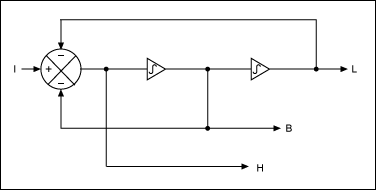We know that the characteristic of an integrator is simply ω0/s. But to demonstrate the principle while simplifying the mathematics, we can assume that both integrators have ω0 = 1 and that their characteristic is simply 1/s. Then we can write equations for each of the integrators in Figure 11:

L = B/s or B = sL

B = H/s or H = sB = s2L

The equation for the summing junction in Figure 11 is simply:

H = I - B - L

If we substitute for H and B using the integrator equations, we get:

s2L = I - sL - L

Or:

s2L + sL + L = I

In which case:

L(s2 + s + 1) = I

Or:

L/I = 1/(s2 + s + 1)

Equation 22 is the classic, normalized, lowpass response. Because B = sL and H = s2L, then:

B/I = s/(s2 + s + 1) and H/I = s2/(s2 + s + 1)

Equation 23 shows, respectively, the classic bandpass and highpass responses.

Thus, one filter provides simultaneous lowpass, bandpass, and highpass outputs. We can create actual filters with real values of ω0 and Q from these equations by building integrators with ω0 ≠ 1 and feedback factors to the summing junction with values ≠1.

In theory you can create higher-order filters by cascading more than two integrators. Some integrated-circuit filters use this approach, but it has drawbacks. To program these filters, you must calculate coefficient values for the higher-order polynomial. Also, a long string of integrators introduces stability problems. By limiting ourselves to second-order sections, we have the advantage of working directly with the ω0 and Q variables associated with each pole.

### Switched-Capacitor Filters

The characteristics of all active filters, regardless of architecture, depend on the accuracy of their RC time constants. Because the typical precision achieved for integrated resistors and capacitors is approximately ±30%, a designer is handicapped when attempting to use absolute values for the components in an integrated filter circuit. The ratio of capacitor values on a chip can be accurately controlled, however, to about one part in 2000. Switched-capacitor filters use these capacitor ratios to achieve precision without the need for precise external components.

In the switched-capacitor integrator shown in Figure 12, the combination of C1 and the switch simulates a resistor.The switch S1 toggles continuously at a clock frequency fCLK. Capacitor C1 charges to VIN when S1 is to the left. When it switches to the right, C1 dumps charge into the integrator's summing node, from which it flows into the capacitor C2. The charge on C1 during each clock cycle is:

Q = C1VIN

Thus the average current transferred to the summing junction is:

I = QfC = C1VIN × fCLK

Notice that the current is proportional to VIN, so we have the same effect as a resistor of value:

R = VIN/I = 1/(C1fCLK)

The integrator's ω0 is therefore:

ω0 = 1/RC2 = C1fCLK/C2

Because ω0 is proportional to the ratio of the two capacitors, its value can be controlled with great accuracy. Moreover, the value is proportional to the clock frequency, so you can vary the filter characteristics by changing fCLK, if desired. But the switched capacitor is a sampled-data system and, therefore, not completely equivalent to the time-continuous RC integrator. The differences, in fact, pose three issues for a designer.

First, the signal passing through a switched capacitor is modulated by the clock frequency. If the input signal contains frequencies near the clock frequency, they can intermodulate and cause spurious output frequencies within the system bandwidth. For many applications this is not a problem, because the input bandwidth has already been limited to less than half the clock frequency. If not, the switched-capacitor filter must be preceded by an anti-aliasing filter that removes any components of input frequency above half of the clock frequency.

Second, the integrator output (Figure 12) is not a linear ramp, but a series of steps at the clock frequency. There may be small spikes at the step transitions caused by charge injected by the switches. These aberrations may not be a problem if the system bandwidth following the filter is much lower than the clock frequency. Otherwise, you must again add another filter at the output of the switch-capacitor filter to remove the clock ripple.

Third, the behavior of the switched-capacitor filter differs from the ideal, time-continuous model, because the input signal is sampled only once per clock cycle. The filter output deviates from the ideal as the filter's pole frequency approaches the clock frequency, particularly for low values of Q. You can, however, calculate these effects and allow for them during the design process.

Considering the above, it is best to keep the ratio of clock-to-center frequency as large as possible. Typical ratios for switched-capacitor filters range from approximately 28:1 to 200:1. The MAX262, for example, allows a maximum clock frequency of 4MHz, so using the minimum ratio of 28:1 gives a maximum center frequency of 140kHz. At the low end, switched-capacitor filters have the advantage that they can handle low frequencies without using uncomfortably large values of R and C. You simply lower the clock frequency.

### Conclusion

This article introduced the concepts and terminology associated with switched-capacitor active filters. If you have grasped the material presented here, you should be able to understand most filter data sheets.